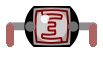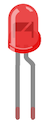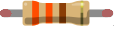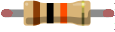# Automatic Dimmer

Educator notes

## Educator Intro

Duration 1,5 - 3h

### Learning Objectives

In this chapter the students will learn…

• … the workflow with simple analog sensors (e.g. light-dependent resistors, potentiometers)
• … to read sensor values from analog pins and to store them into variables
• … to monitor sensor values using serial communication
• … to control devices like LEDs and piezo speakers based on sensor data
• … to map sensor values to a new range
• … to simulate analog signals to control LED brightness

Depending on the final exercise, the students also practice either…

• … observing light and shadow phenomena and using crafts skills to continue developing an Arduino-based instrument (Exercise 1: Improve the Musical Instrument)

or

• … building, coding and testing a burglar alarm prototype (Exercise 2: Burglar Alarm)

### Slides and Lesson Plans

Feel free to use these slides to introduce the third chapter to the students!

There are some speaker notes attached to the slides. To see the speaker notes, click Options > Open Speaker Notes in the bottom panel.

Lesson plan suggestion

TimeWhat?
5-10 minMake sure everyone has the electronics components they need.
SCRUM routine: Students explain to others: 1) what was the last thing they did on the previous lesson and 2) if they had any challenges they need help with. If students are shy to speak when others are listening, you can go and talk with them individually later.
5-10 minIntroduce the third chapter with the slides above.
20-30 min

Students work on Automatic Dimmer.
Go around in the classroom while the students are working and discuss briefly with each student. Talk especially with those who have had problems or seem to lack motivation.

5-10 min

Discuss the challenges so far.
If needed, copy the code from Educator Notes to Arduino IDE, show it on a big screen and go through the parts that have been difficult.

20-30 min

Students work on Noises from the Dark.
Encourage the students to experiment: change the rhythm, make only high or low sounds...
See the Educator Notes for teaching tips and example code.

10 min

Reflection discussion: What has been most interesting? What feels difficult?
Go through the code together if needed.

Introduction to the exercises: give a time limit of eg. 20 minutes!

20-30 minStudents choose and work on an exercise:
1) Improve the Musical Instrument
2) Burglar Alarm
5-10 min

End the lesson (or start the next) by going through a couple of mini projects the students have made.

Total: 90-140 min

## Parts

Do this

Collect these parts. In addition to them you will need an Arduino UNO or Mehackit Board, a USB cable, a breadboard and a bunch of jumper wires.

PartImageDescription
LDR (also called light-dependent resistor, photoresistor, photocell, light sensor)The resistance of an LDR changes with the amount of light. The brighter it gets, the less an LDR resists electric current.
LEDLEDs conduct electricity to one direction only. The longer leg is connected towards a numbered pin (0-13).
Resistor 330Ω (or 220Ω)Resistor resists the flow of an electric current. The value of the resistor is measured in ohms (Ω). The resistance value is coded into the colored stripes (330Ω: orange, orange, brown and gold). You can replace the 330Ω resistor with a 220Ω one for this task.

Resistor 10kΩ (10,000Ω )10 kilo-ohm (10,000 ohm) resistor is used with an LDR. Stripe colors: brown, black, orange, gold

Which direction does current flow in an LED?

Educator notes

As alternative for an LDR you can use a phototransistor, as well. There are different models of phototransistors which vary slightly, so you will need to do an online search to find out how your particular component should be connected to an Arduino (which resistor to use etc.). Phototransistors react faster than LDRs - a good feature in some projects!

## Circuit

Do this

Make a circuit according to the diagram below.

• Connect the LED to pin ~5 and ground (through a 330 ohm resistor)
• Connect one leg of the LDR to 5V row
• Connect the 10 kilo-ohm resistor to ground and to the same row with the other LDR leg
• Connect the row with one LDR and one resistor leg to pin A0

What's special about Arduino's pins marked with the symbol ~?

Educator notes

This is the so-called voltage divider circuit. It's not covered in the student material, as there is already quite a lot of new material introduced in this chapter. Understanding the voltage divider is not considered obligatory in order to proceed with Arduino exercises. But the concept may be related to your curriculum - in case you want to explain the physics behind this technique with your students, here's an explanation to get you started!

Arduino's analog pins can measure voltage changes between 0-5V. Light-dependent resistor, LDR, is a component that has a variable *resistance, *which is something that the Arduino can't measure directly. So you need to be able to measure the change in the voltage of your circuit caused by the light-dependent resistor.

If you only connect one LDR to your circuit, wouldn't it be enough? Just read the sensor values with a wire between the LDR and ground wire, or LDR and voltage wire? Feel free to test it.

If you connect a wire to A0 from between the ground wire and LDR and then check the reading with analogRead, you only get the value 0:

If you connect to A0 between the voltage wire and LDR and check the reading with analogRead, you only get the value 1023.

Why won't these circuits work?

If you try to measure a voltage change with a wire directly connected to ground (which is the case in the first image), the voltage in that point is 0V. And if the wire is connected to the other end, directly to the 5V pin, the voltage measured at that point is 5V.

To measure the actual voltage drop the LDR causes in a circuit, you need the voltage divider:

Now, the point where you measure the voltage change makes more sense! The measuring point is between a fixed resistor (10 kilo-ohms) and a variable resistor (LDR). According to the voltage division rule, the voltage divided between two resistors in series is in direct proportion to the resistance of these resistors.

The voltage division rule is based on this equation:

• V(A0): the voltage measured at pin A0
• V(in): 5 volts, the voltage from pin 5V
• R(fixed): 10 kilo-ohms, the fixed resistor in the circuit
• R(ldr): the resistance of the LDR

The circuit diagram:

## Programming 1: Sensor Values

Do this

Write a new program following the example below.

If needed, check the video or the Arduino Reference to see how to write the commands.

Code example

• Open the serial monitor, cover the LDR and point a light at it. What kind of values do you get?

Here's how you open the serial monitor:

Problems?

• Make sure you have all the parentheses and curly brackets in place!
• Remember: commands must end with a semicolon ( ; )

How does the light-dependent resistor work?
What does the command Serial.begin(9600) do?

Educator notes

Key points:

• new use for a variable: storing sensor values
• using `analogRead`
• checking the LDR values using the serial monitor

You need a delay of 20ms or the values will print too fast.

Code example:

``````//variable for storing the sensor value
int ldrValue;

void setup() {
//start the serial communication:
Serial.begin(9600);
}

void loop() {
//print the LDR value to serial monitor
Serial.println(ldrValue);
//delay of 20ms

delay(20);
}
``````

## Programming 2: LED Brightness

Do this

Continue your code according to the example.

The LED should turn on now (unless you used a brightness value close to 0).

Code Example:

• Give different values to the variable `brightness`. Upload the code to Arduino after every change that you make.

• Also see what happens when you give `ldrValue` to variable `brightness`:

`brightness = ldrValue;`

• If needed, view the video again to see how to write the code. You can also check how to use the commands in Arduino Reference

What happened when you wrote brightness = ldrValue; and uploaded your code to Arduino?

Educator notes

Key points:

• testing different LED brightnesses with `analogWrite`
• using the brightness variable as an argument for `analogWrite`

Code example:

``````//variable for storing the sensor value
int ldrValue;
//create an integer variable called brightness
int brightness;

void setup() {
//start the serial communication
Serial.begin(9600);
//define pin 5 as output
pinMode(5, OUTPUT);
}

void loop() {
Serial.println(ldrValue);
delay(20);
//give brightness a value (0-255)
brightness = 127;
// turn LED on with analogWrite
analogWrite(5, brightness);
}
In the latter part of the coding task, the loop should look like this:

void loop() {
Serial.println(ldrValue);
delay(20);
//give brightness a value (0-255)
brightness = ldrValue;
// turn LED on with analogWrite
analogWrite(5, brightness);
}
``````

With this code the brightness of the LED changes when the LDR is shadowed or when light is pointed to it.

However, especially in bright light the LED will flicker and behave unpredictably.

## Programming 3: Dimmer

Do this

Go to the loop part of your program.

• Map the `ldrValue` to the range 0-255 and store it to the` brightness` variable according to the example.
• Cover the LDR, point a light to it. What happens to the LED?

Code Example

Now, write the last two numbers in the `map` command the other way around:\

Cover the LDR, point a light at it. What happens to the LED now?

EXTRA:

• Open the serial monitor. What are the smallest and biggest LDR values you see if you shadow the LDR or point a light at it?
Change the `map` command like this (the smallest and biggest approximated values are good enough):

This trick will give you a wider brightness range!

Analog pins can record values between 0 and 1023, but you don't always get the whole range of values from a sensor.

Look at this command: `num2 = map(num1, 0, 1000, 200, 400)`.
What is the value of num2 if num1 is 0?
What is the value of num2 if num1 is 1000?
What is the value of num2 if num1 is 500?

Educator notes

Here, the key take away is how to use and adjust the map command.

Flipping the latter range inside the map command will make the dimmer work as intended - the LED will get dimmer when there’s more light:

``````brightness = map(ldrValue, 0, 1023, 255, 0);
``````

Adjusting the range of incoming values inside the map command will make the dimming effect clearer. If the lowest LDR value in the serial monitor is around 100 and the highest around 950, the command would look like this:

``````brightness = map(ldrValue, 150, 950, 255, 0);
``````

The students should investigate the actual minimum and maximum values that they get and use them.